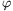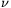Mathematical and Physical Journal
for High Schools
Issued by the MATFUND Foundation
 Already signed up? New to KöMaL?

#Problem A. 574. (November 2012)

A. 574. Let n2 and letbe a polynomial with real coefficients. Prove that if for some positive integer k the polynomial (x-1)k+1 divides p(x) then.

CIIM 2012, Guanajuato, Mexico

(5 pont)

Deadline expired on December 10, 2012.

Solution. For convenience, define the leading coefficient an=1 also.

Lemma. For every polynomial q(y) with degree at most k, we have.

Proof. Let0(y)=1 and letfor. By (x-1)k|p(x), for 0k we haveEvery polynomial with degree at most k can be repesented as a linear combination of the polynomials, sowith some real numbers. ThenTo prove the problem statement, let Tk be the kth Chebyshev-polynomial, and chooseThen, and(In the last step we applied the inequality.)

By applying the lemma,### Statistics:

 3 students sent a solution. 5 points: Ioan Laurentiu Ploscaru. 1 point: 2 students.

Problems in Mathematics of KöMaL, November 2012